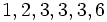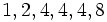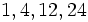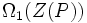# Distribution of normal subgroups in a group

This is a survey article related to:normal subgroup
View other survey articles about normal subgroup

## Introduction

Normality is one of the more frequently encoutered group properties,, and an obvious question is: given a group, which of its subgroups are normal? What structural information about the group correlates with the way the normal subgroups are distributed within the group? This, and other related questions, are explored within this article.

## Numerical feel

### Normal subgroups by order and index for some groups of small order

Group Order Orders of normal subgroups (with multiplicity) Indexes of normal subgroups (with multiplicity) Subgroup structure page
trivial group 1$1$$1$
group of prime order$p$$1,p$$1,p$
cyclic group:Z2 2$1,2$$1,2$
cyclic group:Z3 3$1,3$$1,3$
cyclic group:Z4 4$1,2,4$$1,2,4$ subgroup structure of cyclic group:Z4
Klein four-group 4$1,2,2,2,4$$1,2,2,2,4$ subgroup structure of Klein four-group
cyclic group:Z5 5$1,5$$1,5$
symmetric group:S3 6$1,2,2,2,3,6$$1,2,3,3,3,6$ subgroup structure of symmetric group:S3
cyclic group:Z8 8$1,2,4,8$$1,2,4,8$ subgroup structure of cyclic group:Z8
direct product of Z4 and Z2 8$1,2,2,2,4,4,4,8$$1,2,2,2,4,4,4,8$ subgroup structure of direct product of Z4 and Z2
dihedral group:D8 8$1,2,4,4,4,8$$1,2,2,2,4,8$ subgroup structure of dihedral group:D8
quaternion group 8$1,2,4,4,4,8$$1,2,2,2,4,8$ subgroup structure of quaternion group
elementary abelian group:E8 8$1,2(7),4(7),8$$1,2(7),4(7),8$ subgroup structure of elementary abelian group:E8
alternating group:A4 12$1,4,12$$1,3,12$ subgroup structure of alternating group:A4
symmetric group:S4 24$1,4,12,24$$1,2,6,24$ subgroup structure of symmetric group:S4
special linear group:SL(2,3) 24$1,2,8,24$$1,3,12,24$ subgroup structure of special linear group:SL(2,3)
binary octahedral group 48$1,2,8,24,48$$1,2,6,24,48$ subgroup structure of binary octahedral group
general linear group:GL(2,3) 48$1,2,8,24,48$$1,2,6,24,48$ subgroup structure of general linear group:GL(2,3)
alternating group:A5 60$1,60$$1,60$ subgroup structure of alternating group:A5
symmetric group:S5 120$1,60,120$$1,2,120$ subgroup structure of symmetric group:S5
special linear group:SL(2,5) 120$1,2,120$$1,60,120$ subgroup structure of special linear group:SL(2,5)

## Tools in the study of distribution of normal subgroups

### Lattice of normal subgroups

Further information: Lattice of normal subgroups

Normality is defined as the property of being invariant under all inner automorphisms. Thus, normality is an endo-invariance property, and is hence closed under arbitrary intersections and arbitrary joins. In other words, an arbitrary intersection of normal subgroups is a normal subgroup, and the subgroup generated by any family of normal subgroups is also a normal subgroup.

For full proof, refer: Normality is intersection-closed,Normality is join-closed

Thus, the collection of normal subgroups forms a lattice with the meet operation being intersection of subgroups and the join operation being the join of subgroups (or subgroup generated). This is a sublattice of the lattice of subgroups. Moreover, the sublattice is a complete sublattice, because normality is closed under arbitrary joins and arbitrary intersections.

### Modular lattice

Further information: Modular property of groups, Normal implies modular

Due to the modular property of groups, any normal subgroup of a group is a modular subgroup -- in other words, it is a modular element in the lattice of subgroups. In particular, the lattice of normal subgroups is a modular lattice.

### Arguesian lattice

Further information: Normality is Arguesian

The lattice of normal subgroups of a group is an Arguesian lattice.

## Groups with lots of normal subgroups

### Abelian groups

The property of being normal is an Abelian-tautological subgroup property. In other words, every subgroup of an Abelian group is normal. This follows from the fact that since elements of an Abelian group commute, the only inner automorphism of an Abelian group is the identity map. For full proof, refer: subgroup of Abelian implies normal

Note, however, that an abelian subgroup of a non-abelian group need not be normal. This is because inner automorphisms by elements outside that subgroup may be nontrivial.

### Dedekind groups

A group in which every subgroup is normal is termed a Dedekind group or a Hamiltonian group. Interestingly there can be Dedekind groups which are not abelian. The simplest example is the quaternion group. Moreover, all non-abelian Dedekind groups are closely related to the quaternion group. Further information: Classification of non-abelian Dedekind groups

## Groups with very few normal subgroups

### Simple groups

In every group, the trivial subgroup and the whole group are normal. A nontrivial group for which there are no other normal subgroups, is termed a simple group. Simple groups are opposite to Dedekind groups, and the only possibility for a simple Dedekind group is the cyclic group of prime order.

### Quasisimple groups

A quasisimple group is a perfect group whose inner automorphism group is simple. In a quasisimple group, the only normal subgroups are the whole group and subgroups of the center.

## Behaviour of the lattice of normal subgroups

### Trivial group

The lattice of subgroups of the trivial group is a one-point set.

### Simple groups

Simple groups are the groups where the lattice of normal subgroups is as small as possible: it just has two elements.

### Normal-comparable groups

Normal-comparable groups are groups in which any two normal subgroups are comparable, i.e., one is contained in the other. Some facts about normal-comparable groups:

A somewhat stronger condition than being normal-comparable is being a Jordan-unique group, which is a group that has a unique composition series.

For a (finite) normal-comparable group, the lattice of normal subgroups is a totally ordered set, hence it is graphically represented as a straight line. These normal subgroups form the unique chief series of the group and the length of this series is the chief length.

## Groups of prime power order

### General remarks

Groups of prime power order have a potentially complicated lattice structure for normal subgroups, but this structure still has a number of remarkable properties.

Here are some (related) facts about the bottom end of the lattice of normal subgroups:

• Prime power order implies nilpotent, prime power order implies not centerless
• Prime power order implies center is normality-large, nilpotent implies center is normality-large: In a finite$p$-group$P$, the intersection of the center of$P$ with any nontrivial normal subgroup of$P$ is again a nontrivial normal subgroup.
• Minimal normal implies central in nilpotent, omega-1 of center is normality-large in nilpotent p-group: The minimal normal subgroups are precisely the subgroups of order$p$ contained in the center. The socle, defined as the join of the minimal normal subgroups, is precisely equal to$\Omega_1$ of the center, where$\Omega_1$ is the first omega subgroup.

Thus, the elements just above the trivial subgroup in the lattice of normal subgroups of$P$ are precisely all the subgroups of order$p$ contained in$\Omega_1(Z(P))$. If$\Omega_1(Z(P))$ has$p^d$ elements, there are$[d]_p = 1 + p + p^2 + \dots + p^{d-1}$ such subgroups.

Here are some facts about the maximal subgroups:

• Nilpotent implies every maximal subgroup is normal
• The maximal subgroup of$P$ all contain$\Phi(P)$, the Frattini subgroup of$P$, which is by definition the intersection of maximal subgroups of$P$. It is also the smallest subgroup for which the quotient is elementary abelian. If$|P/\Phi(P)| = p^f$, the number of maximal subgroups (and hence, maximal normal subgroups) is$[f]_p = 1 + p + p^2 + \dots + p^{f-1}$.

The general results are as follows:

• Congruence condition on number of subgroups of given prime power order: There is a normal subgroup of every possible prime power order dividing the order of the group. In fact, the number of such subgroups is congruent to$1$ modulo$p$. Slightly more can be said: the number of p-core-automorphism-invariant subgroups of any given order is congruent to$1$ modulo$p$.
• p-group of automorphisms of p-group is contained in stability group of some normal series: This is a closely related fact, and basically says that we can find chains of maximal length from the identity to the whole group, of normal subgroups invariant under a particular$p$-group of automorphisms.

### Reversal symmetry

For certain kinds of groups, the lattice of normal subgroups is isomorphic to its reverse lattice. Prominent examples include the finite cyclic group, elementary abelian group, and all finite abelian groups (see subgroup lattice and quotient lattice of finite abelian group are isomorphic). However, very few of the$p$-groups, have a lattice of normal subgroups exhibiting reversal symmetry.

For$p = 2$, the smallest examples of$p$-groups whose lattice of normal subgroups does not exhibit reversal symmetry are the dihedral group of order eight and the quaternion group. Both of these groups have lattices that are heavier at the top and lighter at the bottom; this phenomenon is found in extraspecial groups in general. More generally, as observed, the number of minimal normal subgroups depends on the size of$\Omega_1(Z(P))$ while the number of maximal normal subgroups is a function of the size of$P/\Phi(P)$.